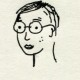# ~Taeyoon

## 28 Jan 2013This was a really fun an learning experience. At first it was a bit frustrating to figure out where to start. However after insightful instructions from Golan, everything was pretty smooth. The code is pretty basic, and it can use much more work. Basicly it’s checking video feed for difference between threshold value and each pixels.If it is darker than the threshold value, the character will jump up (ascend) and if not, it will fall down. I wanted to make the characters rotate when they bounce up, and also to be able to move text from right to left and back, but didn’t get there yet.

Second video has an epic fail near the end.

```PFont f;
String message = ":-@ :-> K@))(*#dfghj:-& k:-::::P:-*&**@!!!!~~®-P*#dfghj:-& k:-::::P:-*&*#dfghj:-& k:-::::P:-*&lL";
// An array of Letter objects
Letter[] letters;
//char[] letters;
import processing.video.*;

int numPixels;
Capture video;

int m;
int n;
int threshold = 95;
int index = 0;

void setup() {
size(640, 480, P2D); // Change size to 320 x 240 if too slow at 640 x 480
strokeWeight(5);
// Uses the default video input, see the reference if this causes an error
video = new Capture(this, width, height);
video.start();
numPixels = video.width * video.height;
noCursor();
smooth();

f = createFont("Arial", 25, true);

textFont(f);

letters = new Letter[message.length()];

// Initialize Letters at the correct x location
int x = 8;
for (int i = 0; i < message.length(); i++) {
letters[i] = new Letter(x, 200, message.charAt(i));
x += 10;
//x += textWidth(message.charAt(i));
}
}

void draw() {

if (video.available()) {

//video.filter(GRAY);
image(video, 0, 0);
float pixelBrightness; // Declare variable to store a pixel's color

for (int i = 0; i < letters.length; i++) {       letters[i].display();       if (mousePressed) {         letters[i].goup();         // letters[i].display();       }        else {         letters[i].shake();         letters[i].check();       }     }   //background(51, 0, 0);     updatePixels();         } } class Letter {   char letter;   // The object knows its original "home" location   float homex, homey;   // As well as its current location   float x, y;   Letter (float x_, float y_, char letter_) {     homex = x = x_;     homey = y = y_;     letter = letter_;   }   // Display the letter   void display() {     //fill(0, 102, 153, 204);     fill(223, 22, 22);     textAlign(LEFT);     text(letter, x, y);     //x+=10;   }   // Move the letter randomly   void shake() {     int m, n;     m = int(x);     n= int(y);     int testValue= get(m, n);     float testBrightness = brightness(testValue) ;     if (testBrightness > threshold) {
x += random(-1, 1);
y += random(5, 10);

if (y > 400) {
y = -height;
index = (index + 1) % letters.length;
}
}
else {
x -= random(-1, 1);
y -= random(20, 26);
}
}

void check() {
float q= textWidth(message);

if (y> 460) {
y = 30;
index = (index +1)%letters.length;
fill(323, 22, 22);
noStroke();
// rect(14, 34, 45, 45);
}
else if (y<30) {
y = 60;
index = (index +1)%letters.length;
fill(223, 22, 22);
noStroke();
//  rect(10, 30, 40, 40);
}

else {
// fill(123, 22, 22);
noStroke();
//  rect(10, 30, 40, 40);
}
}

void goup() {
x -= random(-2, 2);
y -= random(10, 15);
}

// Return the letter home
void home() {
x = homex;
y = homey;
}
}```